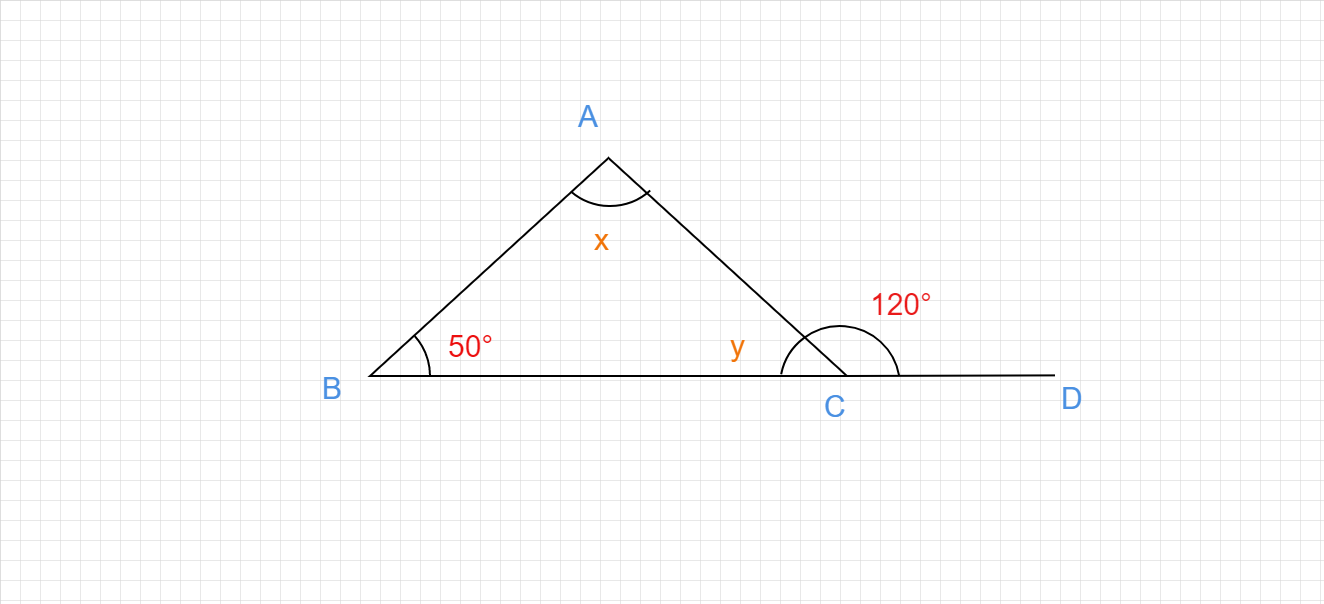"
">

# From the adjoining figure find the values of x and y:"

Given :

The triangle with an internal angle one internal angle 50° and an exterior angle 120° is given.

To do :

We have to find the value of x and y.

Solution :

We know that,

The sum of the angles on a straight line is 180°.

The sum of the angles in a triangle is 180°.

From the figure,

$y+120°=180°$

$y = 180°-120°$

$y = 60°$

$x+50°+y = 180°$

$x+50°+60° = 180°$

$x+110° = 180°$

$x = 180°-110°$

$x = 70°$.

The values of x and y are 70° and 60° respectively.

Updated on: 10-Oct-2022

83 Views